Data & Intelligence

# Graph Model with Gremlin## Graph Theory:

A graph is a pictorial representation of a set of objects where some pairs of objects are connected by links. The interconnected objects are represented by points termed as vertices, and the links that connect the vertices are called edges. Formally, a graph is a pair of sets (V, E), where V is the set of vertices and E is the set of edges, connecting the pairs of vertices.

Graphs are an easy way of representing and visualizing the relationships between many variables.
For example, “Is A dependent on B given that we know the value of C?”

Let’s see how the graph model is implemented on top of the Cassandra database using DSE.

DSE uses the graph structures to store the data with graph model relationships and can process a greater volume of records. The DSE graph is built on top of the DSE database.

## Import/Export Data in DSE Graph:

• By using the command line Utility, we can load the data from CSV, JSON, Text files and queries from JDBC compatible databases.
• Gremlin scripts will insert the data into Graph.
• Graphson Files are JSON formats that can exchange graph data and metadata.

## Datastax Studio:

Datastax studio is a web based graph development tool that helps to create graphs.It consists of three elements:

Vertex:

A Vertex is an object, such as person, location, automobile or anything else you can think of as nouns.

Edge:

An edge defines the relationships between two vertices.

Property:

A key value pair to describe the attribute of either vertex or edge.

Below is the simple graph creation process with DSE graph using gremlin console.

Step 1:

Initial process is to set the environment as Development or Production. Here is the whole process with Development mode.

schema.config().option(‘graph.schema_mode’).set(‘Development’)

schema.config().option(‘graph.allow_scan’).set(‘true’)

Step 2:

For graph, let’s start with creating the vertex as Book, Author and Genre. Then for the vertex,the label should create with proper denotation.

Author Vertex: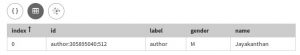Book Vertex:

PavamIvalOruPappaththi = graph.addVertex(label,’book’,’name’,’Pavam, Ival Oru Pappaththi!’,’Year’,1979)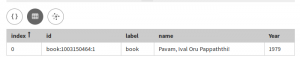##### The Future of Big Data

With some guidance, you can craft a data platform that is right for your organization’s needs and gets the most return from your data capital.

Get the Guide

We can list the vertices for the field which we created below,

g.V().values(‘name’)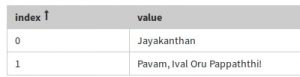Step 3:

Create the edge between the author and book vertices.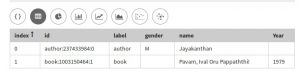We can pull the details of edges we have created,

g.E().hasLabel(‘authored’)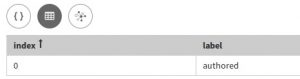Step 4:

Before going to create more records, schema creation and benefits of it are mentioned below,

1. Schema is used to mention the possible properties and the data type in the graph.
2. These properties will be used in the definitions of vertex labels and edge labels.
3. With schema, we can create the index and it plays an important role in retrieval for graph traversal.

Property Keys

schema.propertyKey(‘name’).Text().ifNotExists().create()        // Check for previous creation of property key with ifNotExists()

schema.propertyKey(‘gender’).Text().create()

schema.propertyKey(‘year’).Int().create()

schema.propertyKey(‘timestamp’).Timestamp().create()

After executing the above gremlin code, the property should be defined with a datatype. Here, datatypes are similar with the Cassandra data types. Once the property keys are created, we can proceed with creating the vertex labels and edge labels.

Note: In production environment, creating the vertex labels and edge labels are allowed only after creating the property keys.

 schema.vertexLabel(‘author’).ifNotExists().create() schema.vertexLabel(‘genre’).create() schema.vertexLabel(‘book’).create() schema.edgeLabel(‘authored’).connection(‘author’,’book’).ifNotExists().create() schema.edgeLabel(‘created’).connection(‘author’, ‘genre’).create() schema.edgeLabel(‘type’).connection(‘genre’, ‘book’).create()

Then create the index based on the property field like below,

// Secondary

// Materialized

Secondary Index:

Identify the vertex label and property key for the index, in the vertexLabel() and by() steps, respectively. In the index() step, name the index. The secondary() step identifies the index as a secondary index.

Materialized Index:

Identify the vertex label and property key for the index, in the vertexLabel() and by() steps, respectively. In the index() step, name the index. The materialized() step identifies the index as a materialized view index.

Below is the code related to books and their authors

Below is the final output in the graph format and in keyspace graph view,

Keyspace Graph: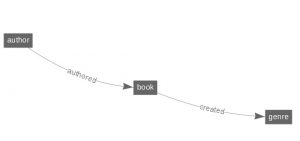Graph: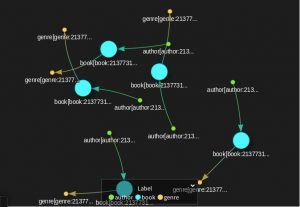Table View: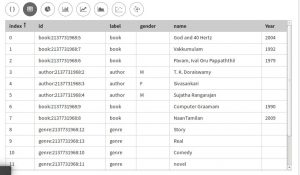This site uses Akismet to reduce spam. Learn how your comment data is processed.